# Color/Tone & eSFR ISO noise measurements

Current Documentation
View previous documentation
View legacy documentation
All documentation versions

including raw (image sensor), chroma, and visual noise
as well as several types of Dynamic Range calculation

 News– Imatest 5.2: Multicharts (the highly interactive module for measuring color accuracy, tonal response, and noise) has been renamed to Color/Tone Setup, and Multitest (the fixed/batch-capable version of Multicharts) has been renamed to Color/Tone Auto. For now the old names will be used for INI file sections. An INI File Monitor captures the contents of INI files (which can be used control Imatest IT runs), and displays them in a web browser table.  Imatest 5.1: Color/Tone Setup and Auto can now measure temporal noise and SNR. With this enhancement, these two new modules have all major features of the older Stepchart and Colorcheck modules.

Color/Tone Setup (formerly Multicharts: Imatest ‘s interactive module for measuring most well-known color and grayscale charts), Color/Tone Auto (formerly Multitest: the batch-capable non-interactive version of Color/Tone Setup), and eSFR ISO (Imatest ‘s module for analyzing ISO 12233:2017 Edge SFR (eSFR) charts) can measure several flavors of noise and Signal-to-Noise Ratio (SNR), including standard pixel noise, chroma noise, ISO 15739 and CPIQ visual noise, Imatest Dynamic Range, ISO 15739 SNR and Dynamic Range, and the intrinsic noise and SNR of image sensors, which is derived from raw images.

### Introduction

Noise is measured for charts with large enough patches to obtain good noise statistics, such as the 24-patch X-Rite Colorchecker or the circular grayscale near the center of ISO 12233:2017 Edge SFR charts. Prior to running any of modules that can analyze noise, you need to select the amount of noise detail to calculate (1. none; 2. elementary; 3. advanced; Visual: a classic tradeoff of detail versus speed; the numbers refer to the table below).

### Settings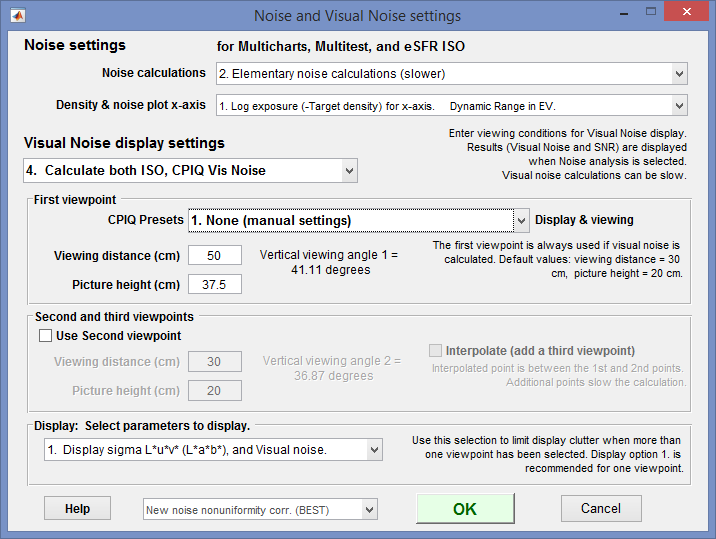Noise and Visual noise settings, opened by any of the above actions

The first setting, Noise calculations, lets you trade off calculation detail and speed. Choices are

 1. No (non-visual) noise calculations (fastest) Use this setting when noise is not needed. 2. Elementary noise calculations (slower) Includes noise types 1-3 and 10-15 from the table below 3. Advanced noise calculations (slower; includes chroma noise) Includes noise types 4-9 (Chroma and CIELAB) from the table below.

The second setting, Density & noise plot x-axis, lets you select the x-axis for noise plots. Choices are

 1. Log exposure (-target density). Dynamic Range in EV. 2. Lux (incident Lux / 10^density). Dynamic Range in EV. 3. Exposure in dB (-10*target density). Dynamic Range in dB. 4. Log exposure. Dynamic Range in Density units 5. F-stops (EV) Density Range in EV  (F-stops)

The third setting, Visual noise display settings, lets you select the visual noise calculation.

 1. No Visual noise calculation 2. Calculate ISO 15739 Visual noise 3. Calculate CPIQ Visual noise 4. Calculate both ISO, CPIQ Vis noise

### To display noise,

 Color/Tone Setup (Multicharts) Click on 11. Noise analysis (not for all charts) in the noise display options located just to the right of the Settings buttons in and just below the Noise/SNR checkbox. Color/Tone Auto (Multitest) Open the Settings window (from the Imatest main window or during a Color/Tone run) and select Plots 8 and/or 9 (on the right). (Yes, Color/Tone allows two noise plots because there are so many options.) eSFR ISO   (run from Rescharts or eSFR ISO Setup) Under Display (on the right) select 16. Noise. Noise Plot (eSFR ISO-only) is available in eSFR ISO Auto.

Twenty-four different noise plots are available. Settings 1-9 and 13-21 measure noise (or SNR) for the grayscale patches-only. Settings 10-13 apply to all patches (including color); these are of particular interest for analyzing sensor noise from raw images.

 1 Noise vs. input density (RGB) Noise in pixels or % of maximum pixel level 2. Simple noise or SNR derived from standard deviation (σ) of pixel levels. 2 Signal/Noise (S/N) vs. input density (RGBY) The luminance (Y-channel) is used for S. 3 SNR (dB) vs. input density (RGBY) SNR (dB) = 20 log10(S/N). 4 Chroma noise vs. input density 3. Industry-standard chroma noise 5 Chroma S/N vs. input density 6 Chroma SNR (dB) vs. input density 7 CIELAB (L*a*b*) noise 3. Experimental: Seems to make sense since CIELAB is approximately perceptually uniform, but has no industry traction. 8 CIELAB (L*a*b*) S/N 9 CIELAB (L*a*b*) SNR (dB) 10 Noise vs. pixel (all patches) 2. For characterizing sensors from RAW (unprocessed or minimally processed) images. 11 S/N vs. pixel (all patches) 12 SNR (dB) vs. pixel (all patches) 13 F-stop (scene-referenced) noise, including Dynamic Range results. 2. Used for Dynamic Range calculations 14 F-stop (scene-referenced) S/N, including Dynamic Range results. 15 F-stop (scene-referenced) SNR (dB), including Dynamic Range results. 16 ISO 15739 Visual noise vs. input density (L*u*v*) V. From the ISO 15739:2013 standard. Includes viewing conditions. 17 ISO 15739 Visual S/N vs. input density (L*u*v*) 18 ISO 15739 Visual SNR (dB) vs. input density (L*u*v*) 19 ISO 15739 Noise and Dynamic Range 2. Also from ISO 15739:2013. Completely independent of visual noise. 20 ISO 15739 Signal/Noise (S/N) and Dynamic Range 21 ISO 15739 Signal-to-Noise Ratio (SNR) in dB 22 CPIQ Visual noise vs. input density (L*a*b*) V. From IEEE CPIQ P1858TM (Camera Phone Image Quality) specification. See Imatest CPIQ 2016 Support. 23 CPIQ Visual S/N vs. input density (L*a*b*) 24 CPIQ Visual SNR (dB) vs. input density (L*a*b*)

Notes:  2., 3.:  Noise calculation must be set to at least 2 =  Elementary or 3 = Advanced.
V.  The ISO 15739 Visual Noise checkbox must be checked.

Here is a typical noise result for a JPEG image of the X-Rite Colorchecker. The plot is for the grayscale patches (bottom row).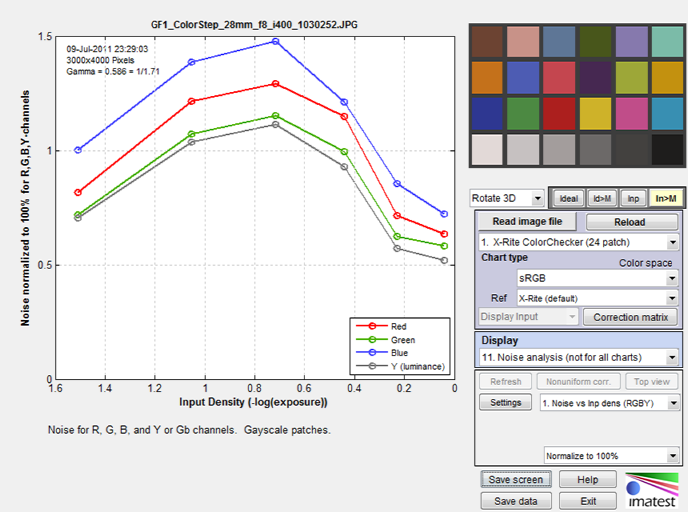Noise vs. input density for grayscale patches. R, G, B, and Y channels.
Panasonic GF1, ISO 400

This plot shows noise, in units of percentage of maximum pixel level, for the R, G, B, and Y (luminance) channels for the grayscale patches (in the bottom row for the Colorchecker). 11. Noise plots is selected in the Display area, and the details of the plot (noise measurements and units) are selected just below Display.

A checkbox below the button allows you to display tonal response (Log pixel level vs. Input density) above the noise (or S/N or SNR (dB)) plot. Here is a result from a JPEG image of the Imatest 36-patch Dynamic Range chart.Log pixel level and Scene-reference (f-stop) noise vs. input density for grayscale patches.

R, G, B, and Y channels. Panasonic G3, ISO 160.

### Noise fundamentals

Noise measurements typically refer to RMS (Root Mean Square) noise, which is identical to the standard deviation of the signal S.

RMS Noise = N =σ(S), where σ denotes the standard deviation.

S can be the signal in any of several channels: R, G, B, Y (luminance, typically 0.2125 R + 0.7154 G + 0.0721 B), or a derived channel such as R-Y or B-Y (both used for chroma noise) or L*, a*, b*, or others. See Multicharts/Multitest/eSFR ISO noise measurements.

 Correcting noise in patches with nonuniform illumination Imatest calculations may deviate slightly from the N =σ(S) formulation because the patch used to measure noise may be unevenly illuminated, and this can result in an increase in measured N not actually caused by random noise itself. To account for this we calculate second order fits to the mean signal value in both the x and y-directions, then subtract the second order fits from the signal prior to calculating noise. Hence Imatest noise measurements may be slightly lower than plain standard deviations.The Imatest calculation can be set in a dropdown menu in the settings windows for modules that calculate noise. We recommend New noise uniformity corr. (BEST), but there is an option (not recommended) for turning the nonuniformity correction off. The old calculation will eventually be removed.

Signal-to-Noise Ratio (SNR) is an important measurement derived from noise and is often considered more important than the noise itself. It is expressed as either a simple ratio (SNR = S/N) or in decibels (SNR(dB) = 20 log10(S/N) (familiar to electrical engineers). In digital cameras noise consists of two parts: fixed noise (sensor dark current noise and thermal (resistive) noise) and shot noise, which increases with the square root of the mean number of photons striking the pixels. This relationship enables us to derive sensor dynamic range from raw (completely unprocessed) noise, as described below.

### Imatest Dynamic range

Dynamic Range (DR) is the range of tones over which a camera responds with good image quality (typically specified by Signal-to-Noise Ratio, SNR) and contrast. It is usually measured in f-stops, which are equivalent to zones or EV (factors of two). It can also be measured in density units, where one density unit = 3.322 f-stops or decibels (dB, where 1 density unit = 20dB).

Imatest has several types of Dynamic Range calculation, which are cross-referenced here.

 Dynamic range from a single transmissive chart image. Stepchart, Multicharts, Mutitest A transmissive chart is such as the Imatest 36-patch Dynamic Range or HDR chart is required because reflective charts do not have sufficient tonal range. Dynamic range from multiple (differently exposed) images Dynamic Range module Uses CSV output of Stepchart of Multitest for several differently exposed images. Usually used with reflective charts, but transmissive charts may also be used. ISO 15739 Dynamic Range from patch with density ≈ 2 Multicharts, Multitest, eSFR ISO Extrapolates Dynamic Range from a single patch with density ≈ 2. Raw sensor Dynamic Range Multicharts, Multitest Fits raw data to an equation from the EMVA 1288 standard, then extrapolates to find DR. The test chart does not have to have as large a tonal range as the DR, but transmissive charts with tonal range ≥ 3 are recommended.

In Multicharts/Multitest, Dynamic Range (based on Scene-Referenced noise or SNR) is defined as density range (in the original scene) over which the scene-referenced RMS noise measured in f-stops (the inverse of the f-stop signal-to-noise ratio, SNR), remains under a specified maximum value. The lower the noise (the higher the SNR, which is the inverse of f-stop noise), the better the image quality. SNR tends to be worst in the darkest regions. Imatest calculates the dynamic range for several minimum SNR levels, from 10 (high image quality) to 1 (low quality).

The most straightforward way to measure a camera’s (or scanner’s) dynamic range is with a transmission step chart illuminated from behind by a lightbox. Reflection step charts such as the Kodak/Tiffen Q-13 or Q-14 are inadequate because their density range of around 1.9 is equivalent to 1.9 * 3.32 = 6.3 f-stops, well below that of digital cameras, though they can be used with multiple exposures and the Dynamic Range postprocessor for Stepchart and Multitest.

### Transmission step chart

Several transmission step charts are listed in Using Stepchart. In this page, we focus on the recommended Imatest 36-patch Dynamic Range and High Dynamic Range charts.

The Imatest 36-patch Dynamic Range test chart, with density steps of approximately 0.1 from base density to base+3.4 (an 11.3 f-stop range), or the 36-patch High Dynamic Range (HDR) test chart, with density steps from 0.1 to 0.3 and a maximum density of at least base+6 (20 f-stops), are strongly recommended. A nearly circular patch arrangement ensures that vignetting has minimal effect on results. A CSV or CGATS reference file with actual densities (required with the HDR chart) is supplied. the chart has an active area of 7.75×9.25 inches on 8×10 inch film.

It also contains slanted edges in the center and corners with 4:1 contrast for measuring MTF. The edges have an MTF50 >= 16 cycles/mm, which is about 3 times better than the best inkjet charts. Registration marks make the regions easy to select, and fully automated region detection is available in Imatest 4.0+. A neutral gray background helps ensure that the chart will be well-exposed in auto exposure cameras (compared to charts with black backgrounds, which are sometimes strongly overexposed).

The standard 36-patch Dynamic Range chart can be used with the Dynamic Range postprocessor to measure dynamic ranges larger than 11 f-stops if several manual exposures are available. Dmax = 3.4 is sufficient for camera phones and digital cameras with small pixel sizes, but high-end DSLRs and HDR security and automotive cameras generally have higher dynamic ranges, which makes them well suited for measurements with either Dynamic Range or the HDR chart (which never requires the Dynamic Range postprocessor).

This chart is produced with a high-precision LVT film recording process for the highest density range, lowest noise, and finest detail.

Lightbox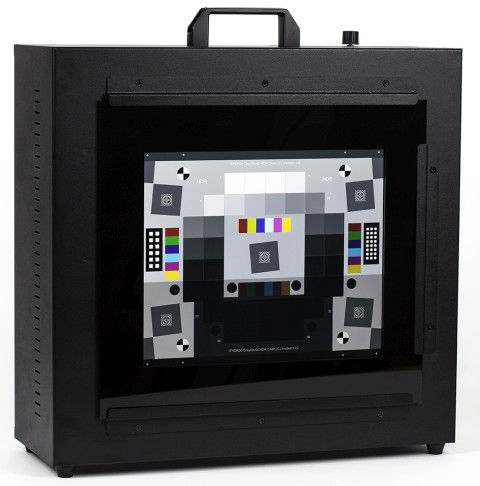You’ll need a lightbox that can evenly illuminate the transmission step chart. 9×12 inches is large enough in most cases. Avoid thin or “mini” models, which may not have even enough illumination. Fluorescent light boxes should have high-frequency ballasts to eliminate flicker. The GLE-10 and GLE-16E, available in the Imatest store are recommended. You can find available lightboxes we offer here.

The best available lightbox is the Imatest LED Lightbox (shown on the right), available from the Imatest store. It has several advantages over the lightboxes mentioned in the previous paragraph.

• Much more uniform illumination.
• High-quality spectral response. The standard version allows you to choose between 3100K, 4100K, 5100K, 5500K, and 6500K color temperature with a Color Rendering Index (CRI) of 97. NIR channels of 850nm and 940nm are available. The box comes with a multi-channel mix mode so you can mix a combination of channels for a continuous range of color temperatures.
• Intensity is adjustable via a hardware knob, WIFI, or USB from 30-10,000 lux: a range of over 300:1, making it suitable for measurements from near-daylight to extremely dim light.

To measure dynamic range,

• Place the chart on the lightbox— or any source of uniform diffuse light. Be sure to block direct light from the lightbox outside of the chart: Stray light can reduce the measured dynamic range; it should be avoided.
• Photograph the chart in a darkened room. No stray light should reach the front of the target; it will distort the results. The surroundings of the chart should be kept as dark as possible to minimize flare light.
• Use your camera’s histogram to determine the minimum exposure that saturates the lightest region of the chart. Overexposure (or underexposure) reduces the number of useful zones. The lightest region should have a relative pixel level of at least 0.98 (pixel level 250 or 255); otherwise, the full dynamic range of the camera will not be detected. If the lightest zone is below this level, and a transmission chart is selected, a Dynamic range warning is issued.
•

For flatbed scanners with transparency units (TPUs, i.e., light sources for transparencies), you can simply lay the chart down on the glass. Stray light shouldn’t be an issue, though there is no harm in keeping it to a minimum.

• Photograph the chart following instructions in Using Multicharts or Using Multitest.

The dynamic range is the difference in density between the zone where the pixel level is 98% of its maximum value (250 for 24-bit color, where the maximum is 255), estimated by interpolation, and the darkest zone that meets the measurement criterion. The repeatability of this measurement is better than 1/4 f-stop.

### Imatest Dynamic range results

Here are results for the Panasonic G3 (a Micro Four-Thirds camera with 3.75 micron pixel pitch) at ISO 160, converted from raw using dcraw with the following settings: Demosaicing: Normal RAW conversion (demosaiced), Output gamma: 2.2, White Balance: Camera, Output color space: 48-bit, Quality: Default.Panasonic G3, ISO 160, Converted with dcraw, run with Multicharts.
Compare with Stepchart results.

The Dynamic Range at low-quality level (scene-referenced SNR = 1) is 9.31 f-stops, decreasing to 5.15 f-stops at high-quality level (SNR = 10). These results are unchanged for 24-bit raw conversion and very close to the results from Stepchart.

The shape of the response curve is a strong function of the conversion software settings. The plot below is for the same exposure, saved as a JPEG file inside the camera. Note that the transfer curve is quite different: it has a “shoulder” in the highlights, which improves pictorial quality by reducing the tendency of highlights to saturate (“burn out”). Dynamic Range is improved due to software noise reduction (absent in the dcraw conversion).Panasonic G3, ISO 160, in-camera JPEG. Note the “shoulder.”
Dynamic range is improved due to software noise reduction.
Compare with Stepchart results.

To convert dynamic range from f-stops into decibels (dB), the measurement normally given on sensor data sheets, multiply the dynamic range in f-stops by 6.02 (20 log10(2)). The dynamic range for low quality (f-stop noise = 1; SNR = 1) corresponds most closely to the number on the data sheets. Measured dynamic range is often lower than specified sensor dynamic range because of lens flare and other factors.

### Chroma noise

Two types of chroma noise display are available.

Standard ISO-based chroma noise.  Displays 4-6 show the noise and SNR for the Y, R-Y, and B-Y channels as well as total noise using two different weightings.  Luminance channel Y = 0.2125 R + 0.7154 G + 0.0721 B). The total weighted noise used in the ISO 12232-2006 and ISO 15739-2013 standards (based on the paper, ISO 12232 revision: determination of chrominance noise weights for noise-based ISO calculation (http://dx.doi.org/10.1117/12.582447) by Sean Kelly and Brian Keelan) is plotted as a solid black line (with ◊ markers). Total weighted noise is σ(D) = [ σ(Y)2 + 0.279 σ(R-Y)2 + 0.088 σ(B-Y)2 ]1/2The older total weighted noise ([ σ(Y)2 + 0.64 σ(R-Y)2 + 0.16 σ(B-Y)2 ]1/2) used in ISO 12232:1998 and 15739-2003 is obsolete and may be deprecated in a future Imatest release.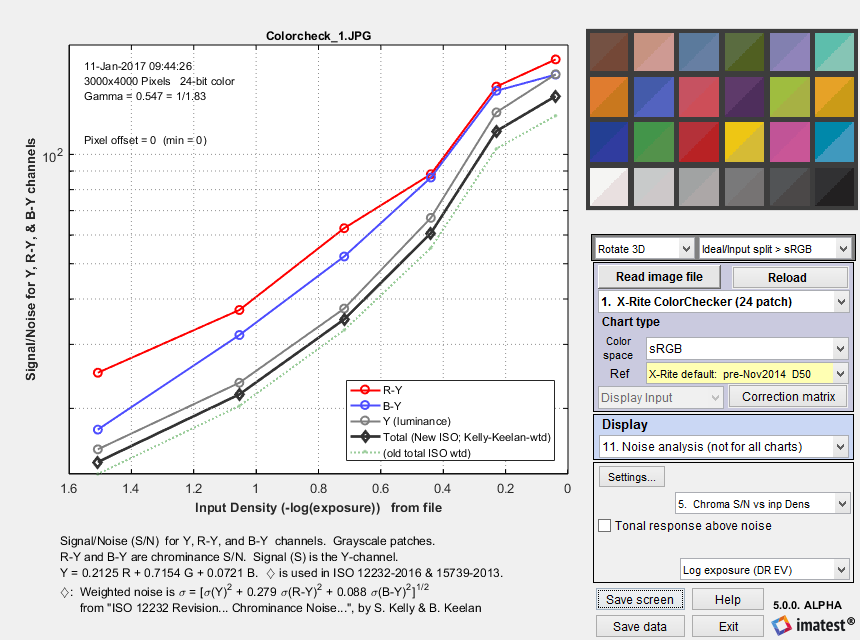Signal/Noise for R-Y and B-Y (chroma) and Y (Luminance) channels,
as well as Total weighted noise from SO 12232-2006 and ISO 15739-2013 (—◊—).

L* a* b* (CIELAB) noise is potentially interesting because CIELAB was designed to be a perceptually-uniform space. Although it is less uniform than intended— which is why color difference metrics like ΔE-2000 were developed— it is still much more uniform than many other representations, such as RGB. CIELAB noise is not a standard measurement. There is very little literature on using CIELAB for noise measurements. even though a* and b* might be a better measure of chroma noise than R-Y and B-Y (above). We are not aware of any weighting factors (for combining L*, a*, and b* into a single number).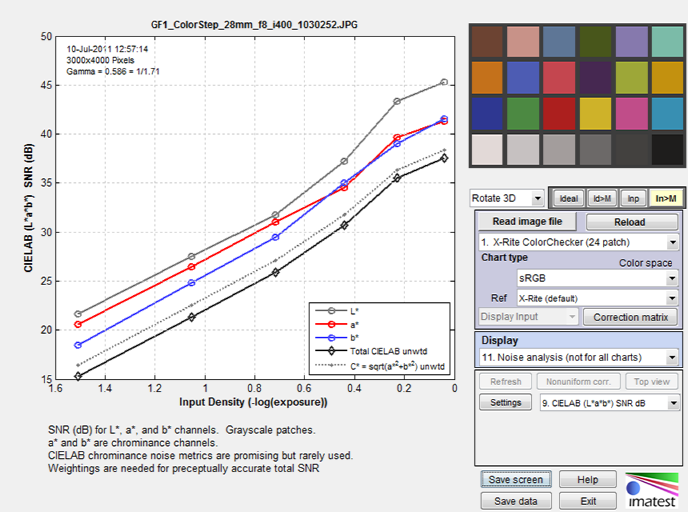Chroma SNR for CIELAB L*, a*, and b*-channels as well as Total noise, unweighted.

### Image sensor (RAW) noise and dynamic range

 Starting with Imatest 4.2, we recommend converting commercial raw files to DNG format, then directly reading them into Imatest for image sensor noise and dynamic range measurements.

A remarkable property of RAW (unprocessed) images is that the noise in a patch of identically-illuminated pixels is a function of the pixel level, independent of color. This property is no longer observable once an image has been processed (demosaiced, white-balanced, noise-reduced, sharpened, etc.).

The equation is quite simple: Noise = (dark noise2 + k pixel level)1/2

For a detailed explanation of this equation we recommend the excellent introduction to the EMVA (European Machine Vision Association) 1288 standard. See www.emva.org/standards-technology/emva-1288/  and also www.emva.org/wp-content/uploads/EMVA1288-3.1a.pdf (the standard itself).

 Using this equation, Imatest can measure sensor noise and dynamic range from unprocessed raw files even if the test chart’s tonal range is less than the sensor’s dynamic range. Although this method sometimes works with low tonal range charts such as the X-Rite Colorchecker, we recommend a chart with a large tonal range (usually a transmissive chart on color film), such as one of the Imatest Dynamic Range charts, for best results.

Raw noise and dynamic range is displayed in the following three noise displays, which are derived from all the patches in the image (color as well as grayscale):

 10 Noise vs. pixel (all patches) 11 S/N vs. pixel (all patches) 12 SNR (dB) vs. pixel (all patches)

To correctly measure sensor dynamic range, the image must be completely unprocessed: it must be un-demosaiced (Bayer RAW) and any black level offset or white level limitations in the raw file need to be removed so that pixel levels range between 0 for black to the maximum for the bit depth (2n-1, e.g., 216-1 = 65535 for bit depth = 16). Since settings have to be exactly correct for valid measurements, we will review the recommended settings for reading a Bayer RAW (un-demosaiced) image.

#### Reading RAW files for sensor noise and dynamic range analysis

Imatest supports two kinds of RAW file.

• Commercial RAW files, from DSLRs and other high-quality consumer cameras. These files are identified by standard extensions (CR2, NEF, RW2, etc.). They contain header data and are usually packed so that m pixels are stored in n bytes. There are normally processed (demosaiced) using dcraw. But for sensor DR measurements, where the file must not be demosaiced, we recommend direct DNG read, as described below.
• Binary RAW files from development systems. These files rarely contain headers, and pixels are usually stored in 1 or 2 bytes. They are processed using Imatest’s Generalized Read RAW function.

#### dcraw front-end for commercial raw files

The method used prior to September 2015 has been deprecated. It involves opening a file in dcraw and selecting the Bayer Raw 16-bit linear preset, which converts the file without demosaicing, sets Gamma = 1.0, and unchecks Auto white level. This method has issues with both the maximum pixel level (rarely 216-1 = 65535) and black level offsets, as described below.

 Black level offset  If there is a pixel offset (a minimum pixel level, AKA a “pedestal” or “floor”) in the raw-converted output, results will be incorrect. A pixel offset can be recognized as a flattening of the log pixel level response (sometimes difficult to distinguish from flare light). Pixel offsets are sometimes powers of 2 (16, 2048, …). Canon CR2 raw files are a case in point. When dcraw converts raw files with normal demosaicing, the pixel offset is removed. Images look good and the expected value of gamma is obtained. But when raw files are converted to 16-bit Bayer raw files without demosaicing, the offset is not removed: Canon CR2 files have an offset of 8192 pixels (applied in Multicharts, after the pixel levels have been shifted) out of a maximum of 65535 (for 16-bit files). The converted files have unexpectedly low contrast and gamma. To obtain correct results, the pixel offset must be entered in the Multicharts or Multitest settings window. The figures below show log pixel vs. log exposure for a Panasonic micro Four Thirds camera. The minimum pixel level is 2016, which appears to correspond to a pixel offset of 2048 bits (we guessed the closest power of 2). Extreme flattening is visible when the offset correction is not applied. There is still some flattening (apparently caused by flare light) when the offset is corrected.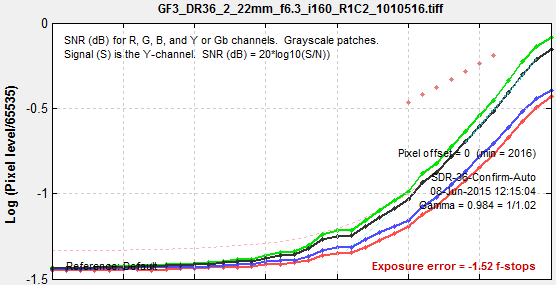#### Reading commercial raw files using DNG

To avoid issues with the black and white levels, we recommend converting raw files (CR2, NEF, RW2, etc.) to Adobe DNG format and reading it directly into Imatest— not using dcraw. This description is taken from Raw Files— Direct DNG (Adobe Digital Negative) read.

Although DNG format offers little advantage when files are demosaiced, it has a significant advantage for measuring image sensor dynamic range, which requires linear undemosaiced (Bayer) raw file data with pixel levels ranging from 0 for pure black to the maximum for the bit format (typically 65535 for 16-bit depth). The problem is that different cameras have different black level offsets (minimum pixel values) and white (saturation) levels, and these values are hard to determine because manufacturers record them with different EXIF tags (if at all). These values are applied to dcraw processing when images are demosaiced, but are ignored when images are not demosaiced (and not saved in the EXIF data). They need to be applied to obtain a valid sensor dynamic range calculation. This can be done automatically for raw files that have been converted to DNG, which is a special type of TIFF file that uses standard tags for linearization and for black and white level correction. See Processing RAW Images in MATLAB for an overview.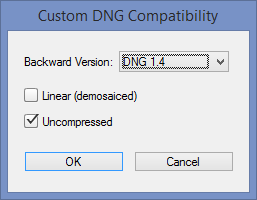• Download and install Adobe’s free DNG converter by going to Adobe’s download page for Windows or Macintosh, and following the instructions. Adobe Digital Negative Converter runs in a GUI window. To use it for undemosaiced files, open it and click Change preferences to open the Preference window, then select Custom… in the Compatibility dropdown window to open the Custom DNG Compatibility window. Linear (demosaiced) should be unchecked and Uncompressed should be checked, as shown on the right.

• To take advantage of this capability, the setting shown on the right, 3. Read DNG files directly then linearize and correct for black level offset, must be selected in the Options II window, which can be opened from a button on the lower-right of the Imatest main window or in the Settings dropdown menu of the Imatest main or Multicharts window.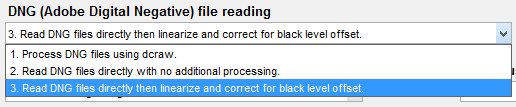When this setting is selected, DNG files read into Imatest have pixel level = 0 for pure black and have a maximum pixel level of 2bit depth-1: (65535 for bit depth = 16), making them well-suited for measuring sensor dynamic range.

• Use the Adobe DNG Converter to convert the raw file to DNG. Operation is simple and straightforward. Note that all files in a folder will be converted, so if you want only a limited number of files to be converted, you’ll need to move them to a separate folder.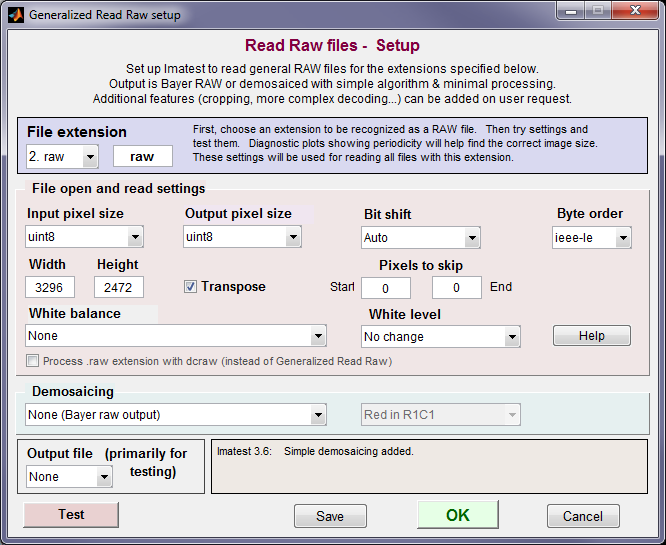Generalized Read RAW settings for “raw” extension.

To use Generalized Read RAW, select Settings, Read Raw Setup from the Imatest, Multicharts, or Rescharts windows. Enter or select an extension name (3 or 4 characters). “raw” is shown here. The settings shown above are for a specific system. You can test the settings by specifying an Output file format and pressing the Test button (lower-left). If you make an incorrect choice of sensor pixel size (Width * Height ≠ total pixels in file) the Test function will display the periodicity and suggest values. For RAW noise analysis, set White Balance to None, Demosaicing to none, White level to No change, and Bit shift to Auto or zero.

#### Interpreting the Bayer RAW (monochrome) file

When used with the recommended settings (above), both the dcraw front-end and Generalized Read Raw produce a monochrome image file that contains Bayer RAW image data. Whenever a monochrome image file is read, the dialog box shown below is opened, and you have to select the image type: Standard monochrome, Bayer RAW (undemosaiced), or Demosaic (convert from Bayer RAW to full color using Matlab’s relatively simple routine).

For measuring image sensor noise, select .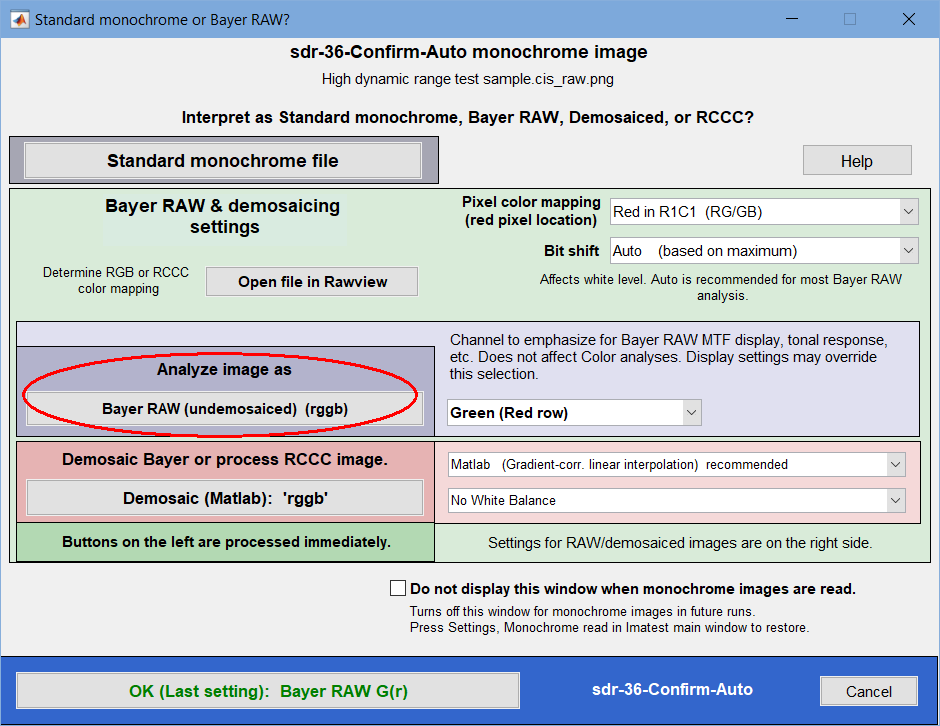Interpretation of monochrome (or Bayer RAW) file.
Select for analyzing sensor noise.

#### Image sensor noise and dynamic range in RAW files

 Linear undemosaiced (RAW) image files have the property that noise is a function of the pixel level-only, independent of color, and can be described by a simple equation. If you have such a file, you can take advantage of this property to measure sensor noise, SNR, and dynamic range, even with a chart that has less tonal range than the sensor being tested. You need to follow the above instructions carefully because this property will be masked if there are black and white level shifts, demosaicing, noise reduction, and the application of a color correction matrix and a gamma/tonal response curve. The figure on the right shows Signal-to-Noise Ratio (SNR) as a function of pixel level for a Raw (RW2) image of an X-Rite Colorchecker, captured with a Panasonic GF1 compact system camera set to ISO 400. The gray line is the fit to the equation, Noise = (dark noise2 + k pixel level)1/2. A sensor dynamic range is calculated, but it’s not entirely reliable because the darkest patch in this chart (which has an optical density range of only 1.5) has SNR = 15dB, much greater than 0dB, which is used for the DR specification. For more robust and reliable results, a transmissive chart (with density range ≥ 3) is recommended.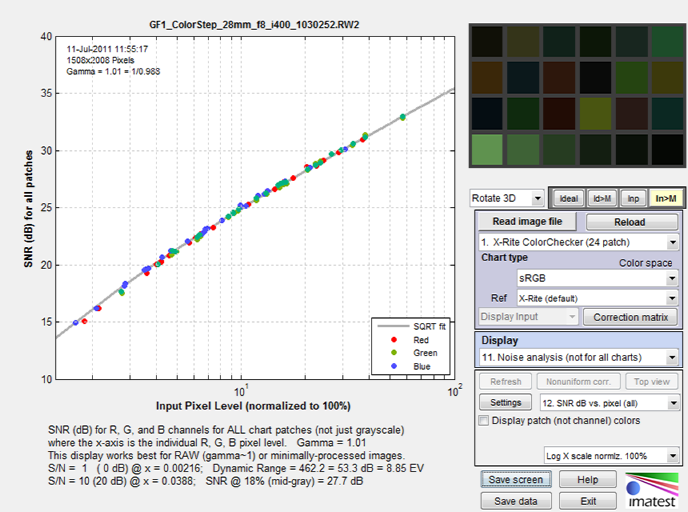SNR (dB) as a function of input pixel level (x-axis displayed logarithmically) SNR(dB) = 20 log10(S/N), where signal S is the mean pixel level of the patch.

Here is the same curve for the new High Dynamic Range chart, acquired with the Canon EOS-6D (a popular full-frame DSLR) set to ISO 100.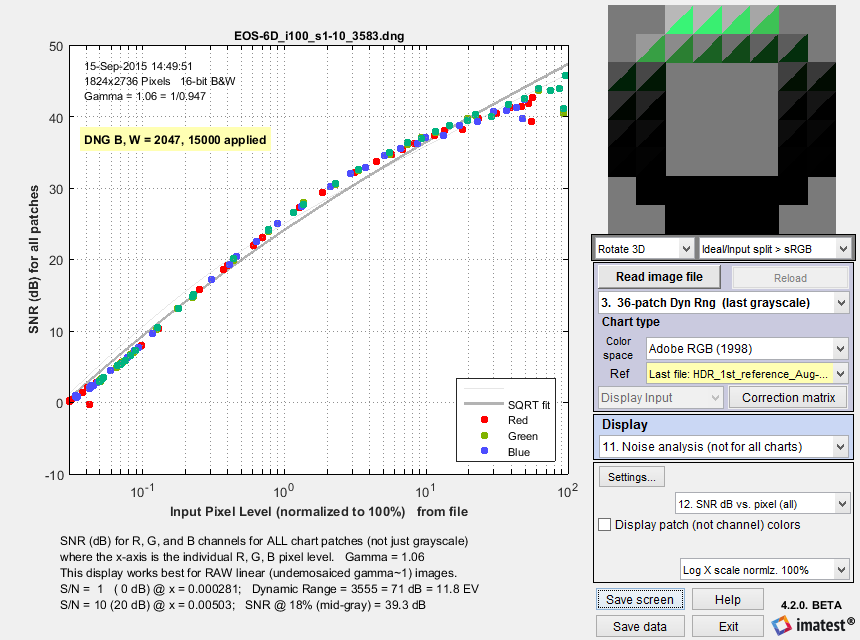SNR (dB) as a function of input pixel level for 36-patch HDR chart, Canon EOS-6D

 This result is extremely reliable because the DNG conversion effectively removed any issues with black and white level offsets and because the chart’s tonal range is extended enough to get down to SNR = 0dB, making the fit (gray line) very trustworthy. The grayscale response (shown on the right) is displayed in other noise plots. (It’s not displayed in the SNR dB vs. pixel (all) plot because it is for all patches, including color patches when they are present.)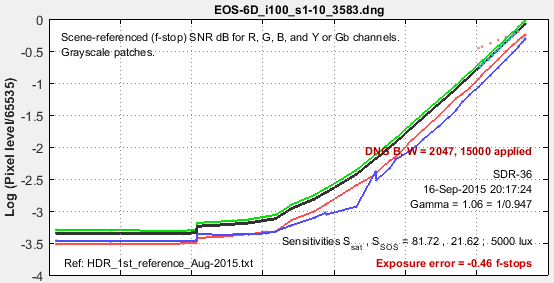Note that the sensor dynamic range is not the same as the camera dynamic range, which includes the effects of lens flare and is also very sensitive to the experimental setup, where reflected light on the front of the test chart can seriously degrade the measurement. Camera dynamic range for the same image is shown on the right: the CR2 raw image was converted to 48-bit Adobe RGB color space (gamma = 1/2.2) with dcraw. The low-quality dynamic range, defined by scene-referenced SNR ≥ 1 = 0dB corresponds most closely to the sensor dynamic range. In this case the numbers (11 and 11.8 f-stops) are close because we are near the threshold of where flare light starts limiting the camera dynamic range. The low-quality dynamic range for the JPEG file of the same capture is still very close at 11.6dB— probably slightly better than the converted CR2 because of software noise reduction. Sensor and camera dynamic range may diverge when there is strong flare light or aggressive signal processing (in the camera). For reference, ISO 15739 dynamic range for this image is 9.28 f-stops. It was calculated using the patch closest to density = 2.0, even though the chart density goes much higher. This measurement is primarily useful for reflective charts, which rarely go much beyond density = 2.0.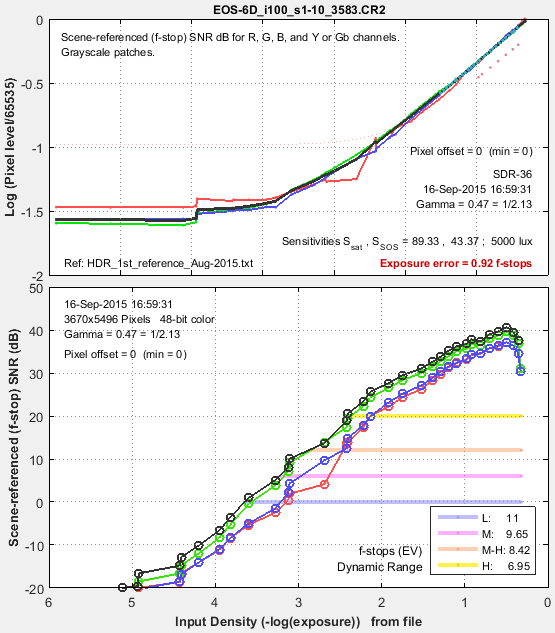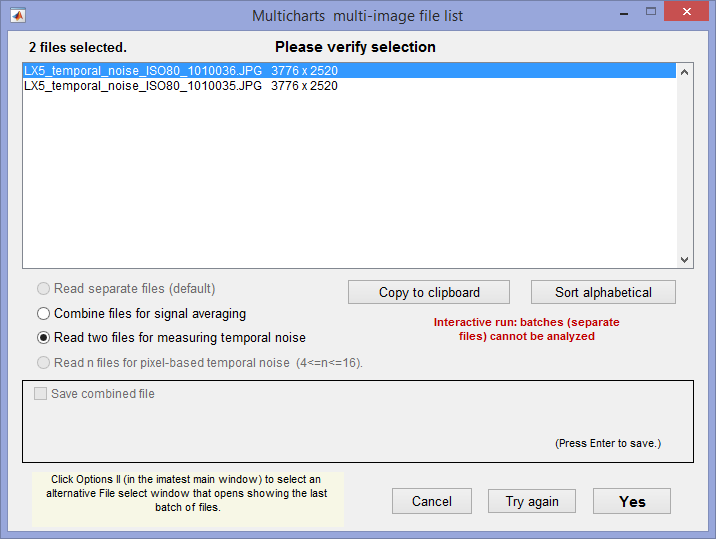### Temporal noise

Temporal noise— noise that varies independently from image to image, with fixed-pattern noise removed— was added to Multicharts and Multitest in Imatest 5.1. It can be calculated by two methods

1. the difference between two identical test chart images (the Imatest recommended method), and
2. the ISO 15739-based method, which where it is calculated from the pixel difference between the average of N identical images (N ≥ 8) and each individual image.

Note that fixed pattern noise can be calculated by selecting Combine files for signal averaging.

(1) Difference method. In any of the modules, read two images. The window shown on the right appears. Select the Read two files for measuring temporal noise radio button.

The two files will be read and their difference (which cancels fixed pattern noise) is taken. Since these images are independent, noise powers add. For independent images I1 and I2, temporal noise is

$$\displaystyle \sigma_{temporal} = \frac{\sigma(I_1 – I_2)}{\sqrt{2}}$$

In Multicharts and Multitest temporal noise is displayed as dotted lines in Noise analysis plots 1-3 (simple noise, S/N, and SNR (dB)).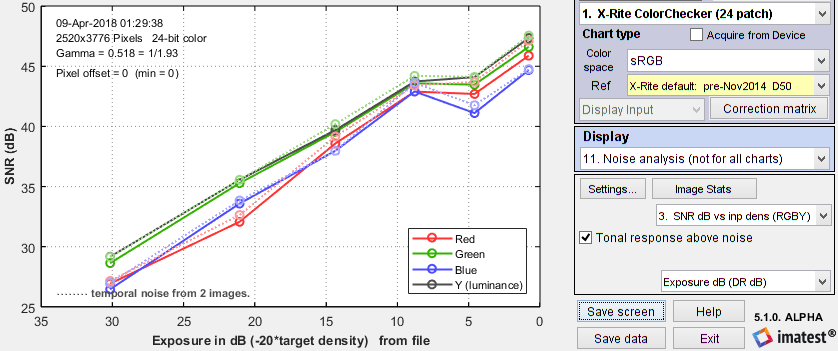SNR (dB) for Colorchecker chart: temporal noise shown as thin dotted lines.

(2) Multiple file method. From ISO 15739, sections 6.2.4, and 6.2.5. Currently we are using simple noise (not yet scene-referred noise). Select between 4 and 16 files. In the multi-image file list window (shown above) select Read n files for temporal noise. Temporal noise is calculated for each pixel j using

$$\displaystyle \sigma_{diff}(j) = \sqrt{\frac{1}{N} \sum_{i=1}^N (X_{j,i} – X_{AVG,j})^2} = \sqrt{\frac{1}{N} \sum_{i=1}^N X_{j,i}^2 – \left(\frac{1}{N} \sum_{i=1}^N X_{j,i}\right)^2 }$$

The latter expression is used in the actual calculation since only two arrays, $$\sum X_{j,i} \text{ and } \sum X_{j,i}^2$$, need to be saved. Since N is a relatively small number (between 4 and 16, with 8 recommended), it must be corrected using formulas from Identities and mathematical properties in the Wikipedia standard deviation page.  $$s(X) = \sqrt{\frac{N}{N-1}} \sqrt{E[(X – E(X))^2]}$$.

$$\sigma_{temporal} = \sigma_{diff} \sqrt{\frac{N}{N-1}}$$

We currently recommend the difference method (1) because our experience so far has shown no advantage to method (2), which requires many more images (≥ 8 recommended), but allows fixed pattern noise to be calculated at the same time. There is a detailed comparison of the methods in Measuring Temporal Noise.

### ISO 15739 and CPIQ Visual noise

Imatest 4.0+ includes a calculation of ISO 15739 visual noise as specified in Appendix B of the ISO 15739:2013 standard. And Imatest 4.4+ includes a calculation of visual noise from the IEEE CPIQ P1858 (Camera Phone Image Quality) 2016 specification, which is based on ISO 15739 with some added details. These are relatively complex calculations that include the effects of the human visual system. It is performed for all grayscale patches for up to three viewing conditions (viewpoints), each of which is specified by viewing distance and display (picture) height. The vertical display angle (in degrees) is

Viewing angle = 360/pi*atan(.5*picture height/viewing distance)

We summarize the calculation in the table below. Details can be found in the ISO 15739:2013 standard, Appendix B and in the IEEE CPIQ P1858 (Camera Phone Image Quality) 2016 specification.

 ISO CPIQ Step Description B.2.1 D.1 D.2 RGB to XYZ(E) Convert RGB to XYZ values for illuminant E, typically by converting RGB to XYZ(D65), then converting from XYZ(D65) to XYZ(E). Though the standard specified sRGB color space (the Windows/internet standard), Imatest can work with any color space. See en.wikipedia.org/wiki/CIE_1931_color_space. B.2.2 D.4 XYZ(E) into opponent space AC1C2 A relatively straightforward matrix operation. B.2.3 Discrete Fourier Transform Transform A, C1, C2 into Fourier space (cycles/pixel). B.2.4 D.6 Apply the contrast sensitivity function (CSF) There are three functions: for A (identical to luminance Y), C1, and C2. Viewing conditions are used here (f in cycles/degree is calculated from the vertical pixel count and angle). D.7 Apply Display/Printer MTF. D.8 High Pass FIlter (HPF) Cutoff < 1 cycle/degree. DC (calculated prior to the filter) is restored after the filter. B.2.5 Inverse Fourier Transform Back to A, C1, C2. B.2.6 D.10 Opponent space AC1C2 into XYZ(E) A relatively straightforward matrix operation. B.2.7 XYZ(E) to XYZ(D65) Another relatively straightforward matrix operation. B.2.8 XYZ(D65) to L*u*v* CIELUV (L*u*v*) is a relatively perceptually uniform color representation that remains relatively unfamiliar. L* is nonlinear: proportional to brightness to the 1/3 power. Note that this is a filtered space (not plain L*u*v*). D.12 XYZ(D65) to L*a*b* (CIE Lab) Numbers seem to be similar to the L*u*v* numbers from ISO 15739. B.2.9 Standard deviation for each grey patch The noise for each (L*, u*, v*) channel is the standard deviation of the levels for the patch. B.2.10 Weighted sum representing the visual noise V = σL* + 0.852 σu* + 0.323 σv* D.13 Objective noise (CPIQ) Ω = log10[1 + 23.0 σ2(L*) + 4.24  σ2(a*) – 5.47 σ2(b*) + 4.77 σ2(L* a*)

Rube Goldberg would have been impressed. His spirit is with us.To adjust visual noise settings

• In Multicharts, click Settings, ISO speed, noise, mxn chart… Then click Visual noise settings.
• In Multitest, click Setup (to the right of Multitest… in the Imatest main window), then click Noise & Visual noise settings.
• In eSFR ISO (run from Rescharts or eSFR ISO Setup), click the More settings button, then the eSFR ISO Noise settings button, or click on the Settings dropdown menu, Noise & Visual noise settings…

These selections open the Noise and Visual noise window, shown above, which allows you to select up to three viewpoints (viewing positions based on distance and picture height, both in cm).

To obtain visual noise results, the viewing distance and picture height in cm for the first viewpoint is required. The viewing angle is calculated from the distance and height.

If these numbers have been entered you can select a second viewpoint, and specify the second viewing distance and picture height. If a second viewpoint has been entered you can select a third, which will have distance and picture height exactly half-way between the first and second viewpoints.

Because visual noise is expressed as the standard deviations of the L*, u*, and v* channels, whose scaling is unfamiliar to most engineers and scientists, Imatest offers three displays of visual noise: one of the noise itself and two of signal-to-noise ratios (expressed as a fraction and as decibels (dB)).

16. ISO 15739 Visual Noise (L*u*v*)
17. ISO 15739 Visual S/N (L*u*v*) Signal-to-Noise Ratio, as a fraction
18. ISO 15739 Visual SNR dB (L*u*v*) Signal-to-Noise Ratio in decibels (dB) = 20*log10(fraction)

We present two examples. The first is for an X-Rite Colorchecker for three viewpoints. All results are presented. (The plot is somewhat cluttered, but still readable).Visual SNR (Signal / Noise) for three viewpoints for an X-Rite Colorchecker.
On the y-axis, 101 corresponds to 20 dB; 102 corresponds to 40 dB.

The second example shows L* noise (σL*) and total visual noise (V) for the same three viewpoints for the Imatest 36-patch Dynamic Range chart. The absolute numbers are somewhat difficult to interpret due to their unfamiliarity: that’s why Imatest also displays Signal-to-Noise Ratios (as fractions and in dB). Noise levels for input densities above (to the left of) 2.0 or 2.5 have little meaning because the image is nearly black (though these levels may be increased by tone-mapping or digital “dodging”).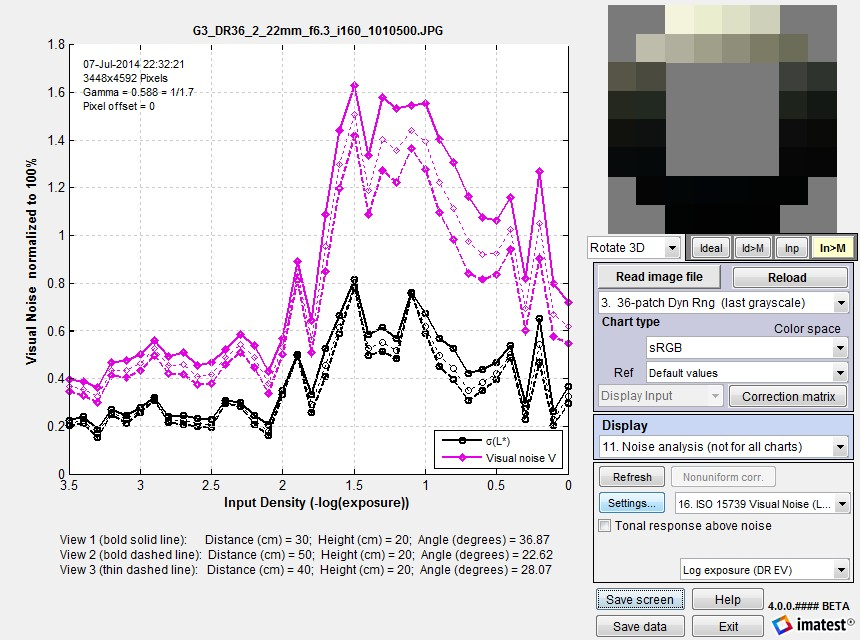Visual noise for three viewpoints for the Imatest 36-patch Dynamic Range chart.

### ISO 15739 SNR and Dynamic Range

The ISO 15739 Dynamic Range (DR) calculation allows DR to be estimated from a patch with density = 2.0, which is available on many semigloss reflective test charts (though density = 2— where 1/100 of the incident light is reflected— cannot be achieved with matte media.

#### Notes on ISO 15739 SNR and Dynamic Range calculations

• These calculations, which are described in sections 6.2 and 6.3 and Annex D of the ISO 15739:2013 Standard, are completely independent of the ISO 15739 Visual Noise measurements, described in Annex B.
• Imatest’s ISO 15739 SNR calculation is primarily for total SNR (§6.2.3). Temporal noise and SNR have been added in Imatest 5.1. There are two calculation methods:  (1) from the difference of two images (divided by √2) and (2) from multiple images (typically 8), following ISO 15739. We recommend method (1) (difference of two images), which is faster and equally accurate. Fixed pattern noise can be measured by averaging at least 8 identical images (by reading multiple images and specifying signal averaging).
 The definition of Signal-to-Noise Ratio (from §6.2.3-6.2.5 and Annex D of the ISO 15739 Standard) is SNR = Q = g L / σ where  σ = noise in pixels, L = luminance (linear), and g is the first derivative of the OECF (the pixel level I vs. luminance curve). g = dI/dL        Luminance L is derived from test chart patch density d (which is equal to (-)log exposure): L = 10–d. The numeric formula for calculating g is given in Annex D. (Pixel level I and Luminance L are smoothed prior to calculating g, with a kernel of 3 or 5 points for <7 or ≥7 patches, respectively.) Though it isn’t obvious from the standard, L must be scaled (multiplied) by reference luminance Lref, as defined in §6.2.2. The definition of Lref is not entirely self-consistent, but it appears that Lref is the luminance that corresponds to a linear pixel level of 91% of the clipping level. For gamma-encoded images with bit depth N, where gamma (γ) is measured from the grayscale patches in the image, this corresponds to a pixel level of Iref = (2N-1)*0.91γ . For standard color space (sRGB) images where γ ≅ 1/2.2 = 0.4545 and N = 8, Iref = 255*0.91(1/2.2) = 244.3     This is close enough to the pixel value of 245 cited in the standard. If the maximum grayscale pixel level is greater than Iref, Lref can be found by interpolation. If the maximum grayscale pixel level is lower than  Iref,  the pixel curve is extended using the measured value of gamma, to find Lref. SNR (Q) is reported at a luminance of 0.13*Lref. This SNR value and the corresponding input density are reported in the lower plot as ISO SNR = Q = … @ Inp Density = … Dynamic range is defined as DR = Lsat/Lmin where Lsat is the saturation luminance (we take the luminance where pixel level = 0.995 of saturation), and Lmin is the luminance where SNR = 1. Since this low SNR level may not be reached (especially with reflective charts) the standard allows Lmin to be estimated (by extrapolation) as Lmin = σ(d=2)/g(d=2). Though we trust this calculation less than a direct measurement, we have found that is it very close in practice. In the plot below (for a JPEG image from the Panasonic G3 at ISO 160), DR(d=2.04) = 3.31 density units; DR(SNR=1) is not measured because the minimum SNR > 1. The Imatest (1/f-stop) scene-referenced DR, shown above (plot 14), is approximately 3.5 (SNR comes very close to 1).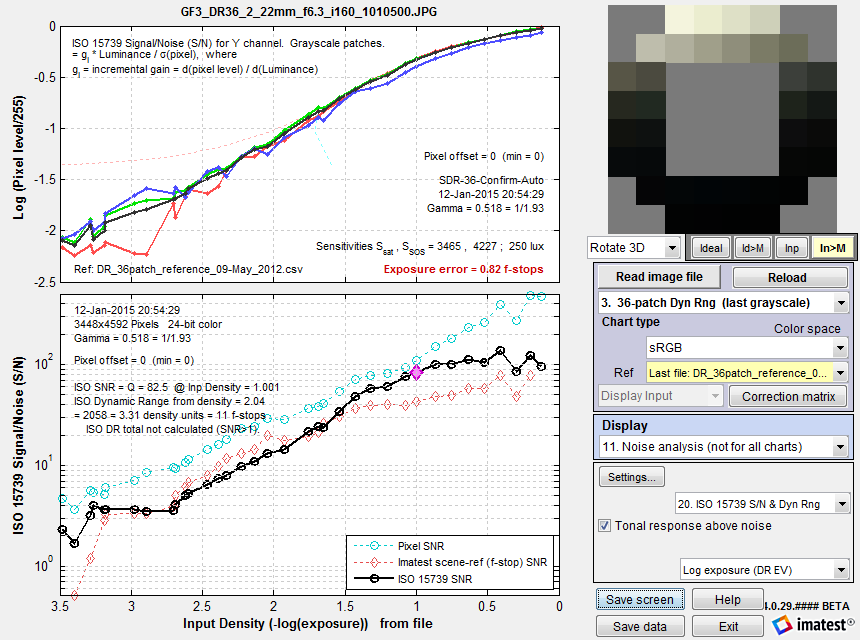ISO 15739 SNR (S/N) for the Imatest 36-patch Dynamic Range chart.
Plain pixel S/N (plot 2) in Cyan; Imatest (f-stop) scene-referenced S/N (plot 14) in Red.

When ISO 15739 SNR is displayed in Multicharts, Multitest, or eSFR ISO, it is compared with the older Imatest f-stop-based calculation. Dynamic Range results tend to be fairly similar. The ISO calculation has two advantages. 1. It conforms to a standard and 2. it allows Dynamic Range to be calculated from SNR for a patch with density near 2— which is achievable with (semigloss) reflective media. This calculation uses extrapolation, which is less direct than finding the density where SNR = 1 (which generally requires transmissive media or several exposures). But in our experience, it produces useful results that are close enough to the direct measurement for practical work, i.e., for comparing different cameras in a standardized way.

#### F-stop noise and scene-referenced SNR

Scene-referenced SNR is the basis of Imatest dynamic range measurements.

#### F-stop noise and scene-referenced SNRThe human eye responds to relative luminance differences. That’s why we think of exposure in terms of zonesf-stops, or EV (exposure value), where a change of one unit corresponds to a factor of 2 change in exposure.The eye’s relative sensitivity is expressed by the Weber-Fechner law,

Δ≈ 0.01 L  –or–  ΔL/L ≈ 0.01

where ΔL is the smallest luminance difference the eye can distinguish. This equation is approximate; effective ΔL tends to be larger in dark areas of scenes and prints due to visual interference (flare light) from bright areas.

Expressing noise in relative luminance units, such as f-stops, corresponds more closely to the eye’s response than standard pixel or voltage units. Noise in f-stops = Nf-stop is obtained by dividing the noise in pixels by the number of pixels per f-stop. (We use “f-stop” rather than “zone” or “EV” out of habit; any of them are OK.) Note that 1 f-stop = 0.301 Optical density units = 6.02dB (decibels) = log2(luminance).

Also note that Nf-stop is scene-referenced noise in (logarithmic) units of f-stops, and must be distinguished from linear scene-referenced noise, Nscene-ref, which has the same linear units as luminance (the scene signal).

 $$\text{F-stop noise } = N_{f-stop} = \displaystyle \frac{N_{pixels}}{d(\text{pixel})/d(\text{f-stop})} = \frac{N_{pixels}}{d(\text{pixel})/d(log_2 \text{luminance})}$$           $$= \displaystyle \frac{N_{pixels} \times ln(2) \times \text{luminance}}{d(\text{pixel})/d(\text{luminance})} ≅ \frac{N_{pixels} \times \text{luminance}}{d(\text{pixel})/d(\text{luminance})}$$ where Npixels is the measured noise in pixels and $$d(\text{pixel})/d(\text{f-stop})$$ is the derivative of the signal (pixel level) with respect to scene luminance (exposure) measured in f-stops = log2(luminance). We used $$d(log_a(x)) = dx / (x \, ln(a))$$. ln(2) = 0.6931 has been dropped to maintain backwards compatibility with older Imatest calculations. Noting that luminance (exposure) is the signal level of the scene,      $$\displaystyle \text{Scene-referenced noise} = N_{scene-ref} = \frac{N_{pixels}}{d(\text{pixel})/d(\text{luminance})} ≅ \frac{N_{f-stop}}{\text{luminance}}$$       $$\displaystyle \text{Scene-referenced Signal-to-Noise Ratio} = SNR_{scene-ref} = \frac{\text{luminance}}{N_{scene-ref}} = \frac{1}{N_{f-stop}}$$ Displays in Stepchart, Multicharts, and Multitest offer a choice between f-stop noise or Scene-referenced SNR (expressed as a ratio or in dB). Note that SNRscene-ref decreases as the slope of the tonal response curve decreases (often the result of flare light in dark patches).

The above-right image illustrates how the pixel spacing between f-stops (and hence d(pixel)/d(f-stop)) decreases with decreasing brightness. This causes f-stop noise to increase with decreasing brightness, visible in the figures above.

Since f-stop noise and scene-referenced SNR are functions of scene luminance, largely independent of image signal processing and fogging from flare light, they are an excellent indicators of real-world camera performance. They are the basis of Imatest Dynamic Range measurements.